Start
Home

# Grade 5 Gap Analysis - Mathematics

#### The number line shown below has a midpoint of 680. Use it to answer question 4.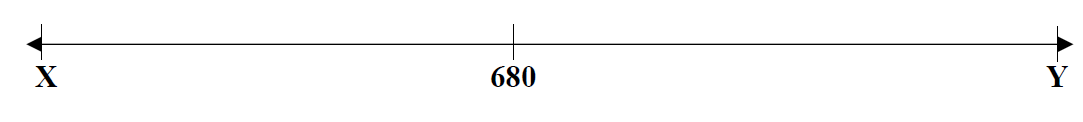#### The diagram below shows 0.47 of a grid shaded. Use the grid to answer question 6.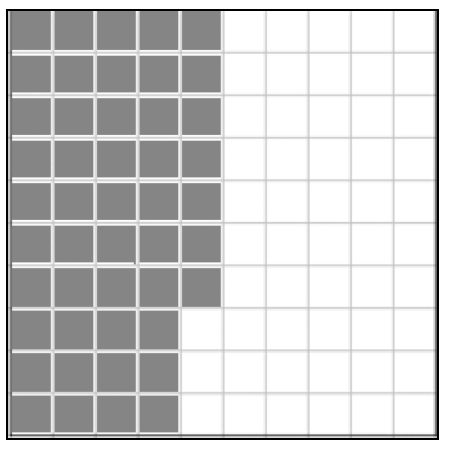#### 8. Which of the following is TRUE about $51.32 × 9? Correct Answer: #### The number sentence below shows a division problem. Use it to answer question 9.42 ÷ 7 = 6 #### 9. Which of the following division problems has the same quotient as the number sentence given? Correct Answer: #### 10. Jason is planning a 7-day long road trip. The total distance to be covered is 567 km. He is planning to drive the same number of kilometres each day. How many kilometres will Jason travel each day? Correct Answer: #### Examine the two number sentences below. The box (□ ) represents the same digit in both sentences. Use the number sentences to answer question 11.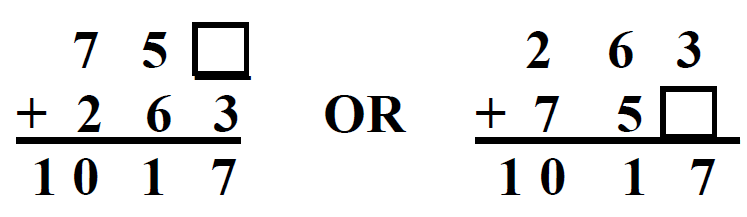#### 11. Which digit should be placed in the box (□) to make both number sentences TRUE? Correct Answer: #### 12. Which pair of decimals has a sum that is greater than 1 and a difference that is less than 1? Correct Answer: #### Ron wants to break$92.50 into two parts in different ways. The table below shows what he has come up with so far. Use the table and this information to answer questions 13 and 14.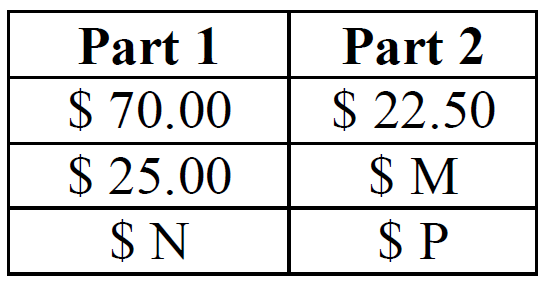#### A list of fractions is listed in the table below. Use them to answer question 15.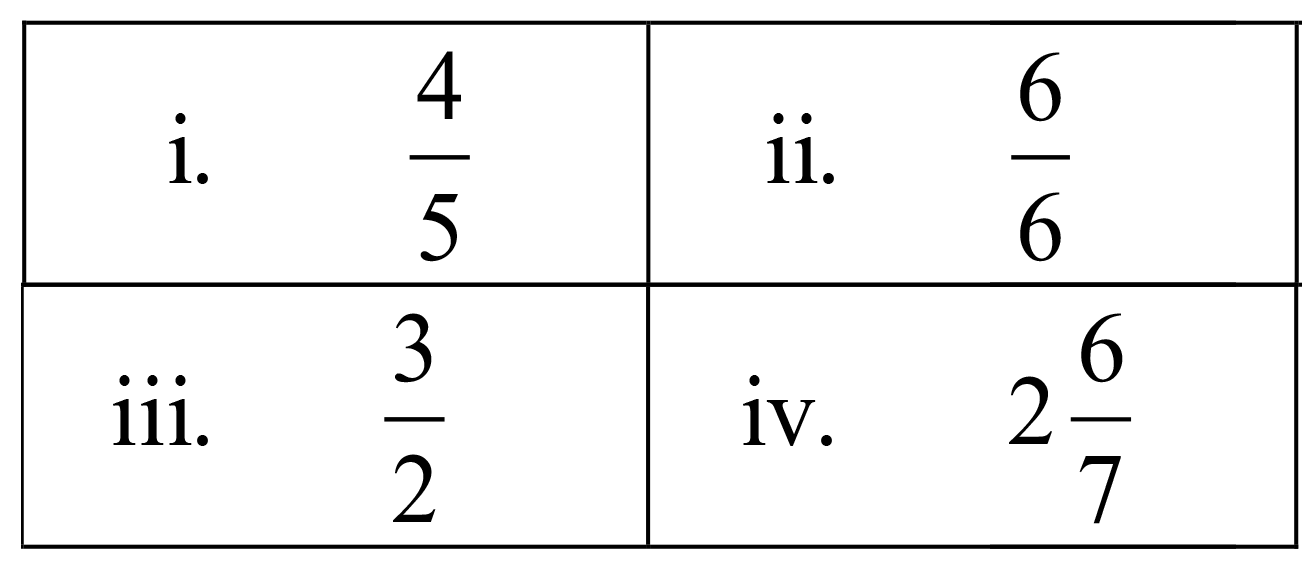#### The diagram below shows a school bus. The school bus can hold a maximum of 8 students. It makes a certain number of trips to town. For each trip, the school bus is no more than $$\frac{7}{8}$$ full. Use this information to answer question 20.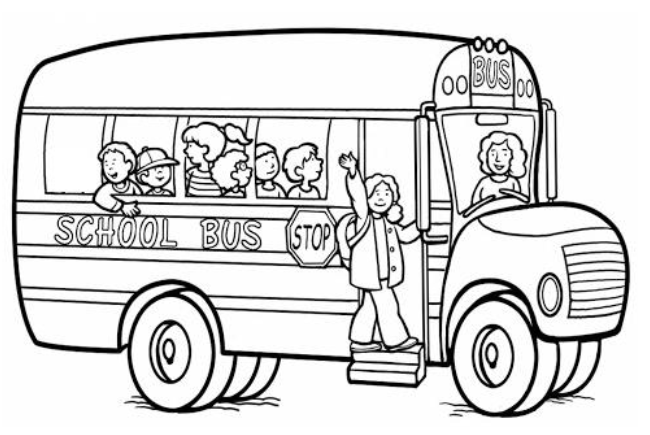#### The diagram below shows a clock. Use it to answer question 22.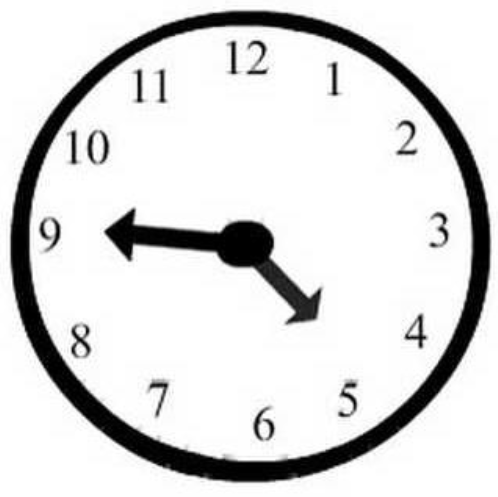#### Examine the shape and its dimensions below. Use it to answer question 28.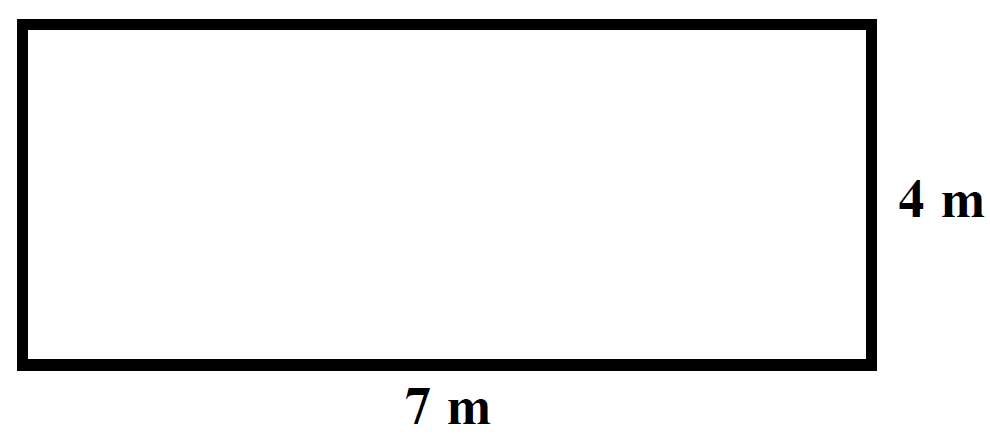#### Three rays are used to make the triangle shown below. Use the triangle to answer question 30.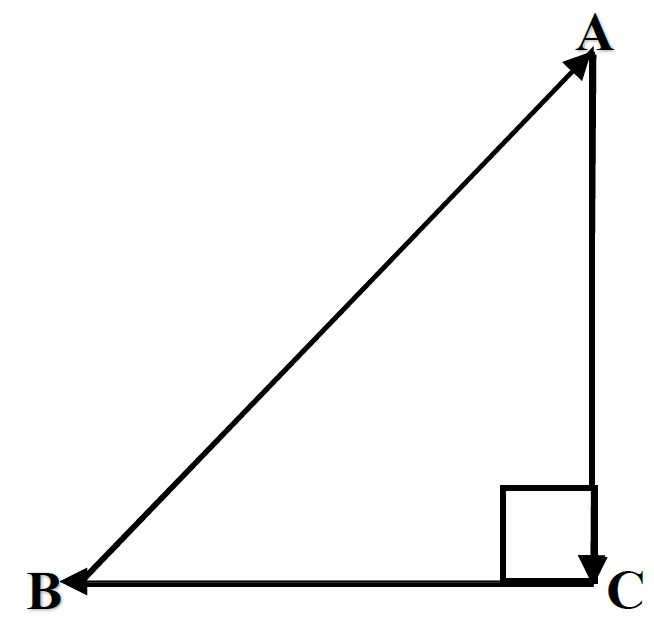#### The diagram below shows an outline of a playground. Use it to answer question 31.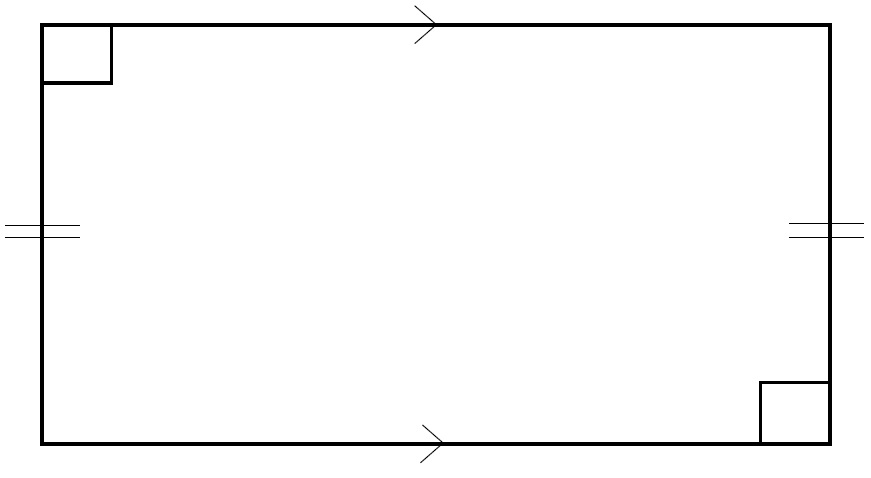#### Two shapes are shown below: Shape G and Shape H. Shape H represents the position of Shape G after it has been flipped. Use this information to answer question 35.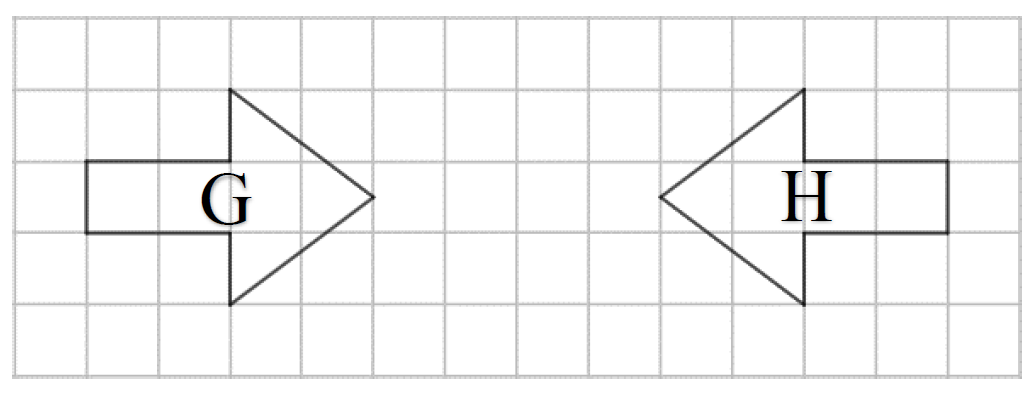#### An angle is shown below. Use it to answer question 37.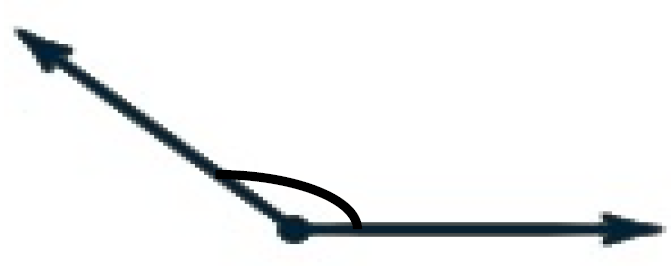#### Examine the growing pattern below. Use it to answer question 41.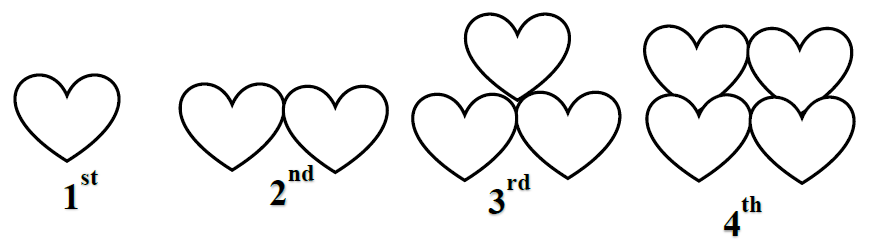#### Some people were asked to select their favourite type of movie. The bar graph below shows their responses. Use it to answer questions 42.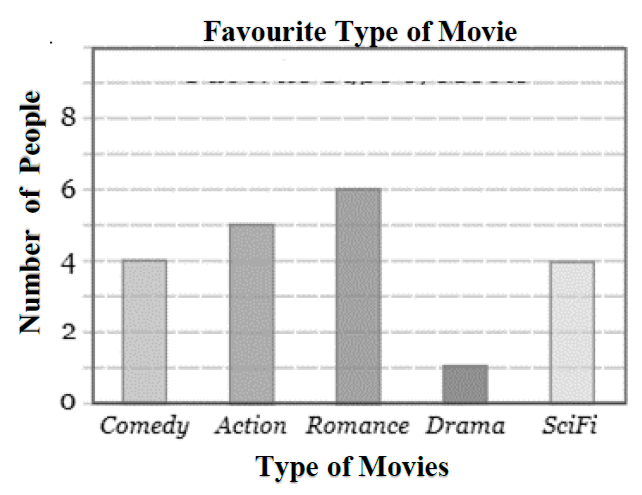#### Some people were asked to select their favourite type of movie. The bar graph below shows their responses. Use it to answer questions 43.#### The table below shows the results of a grade 4 class survey on the students’ favourite colour. Use it to answer question 44.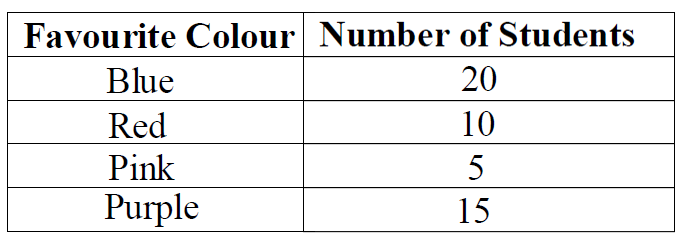#### The line graph below shows the amount of rainfall in a town over four months. Use it to answer question 45.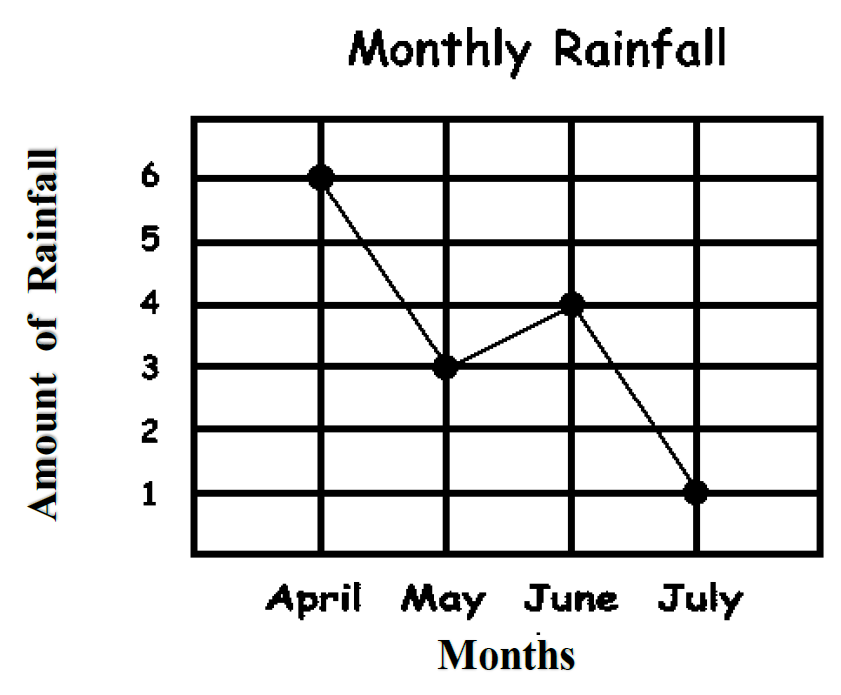#### The picture below shows a fence. The fence is made of pieces of chains and sticks. Two sticks are used to join one piece of chain. Use the picture and this information to help you answer question 49.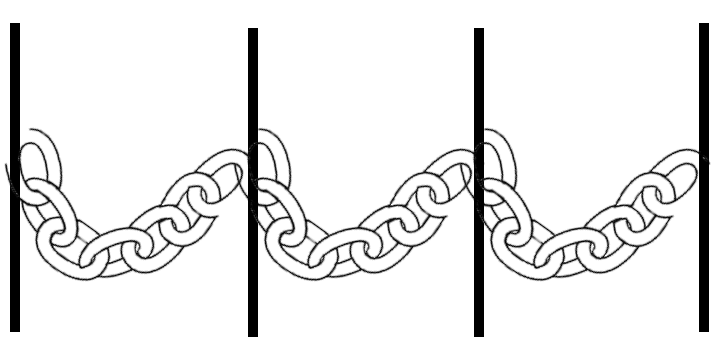#### The diagram below represents the ages of three children: Susan, Carl and Jane. Use it to answer question 50.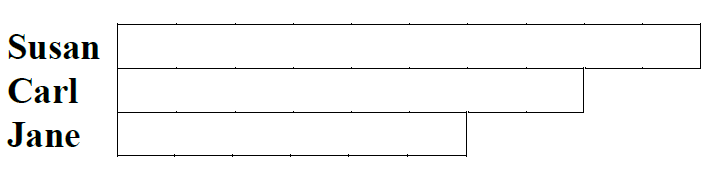#### The table below shows the number of coins four boys collected over a four-week period. Use this information to answer question 53.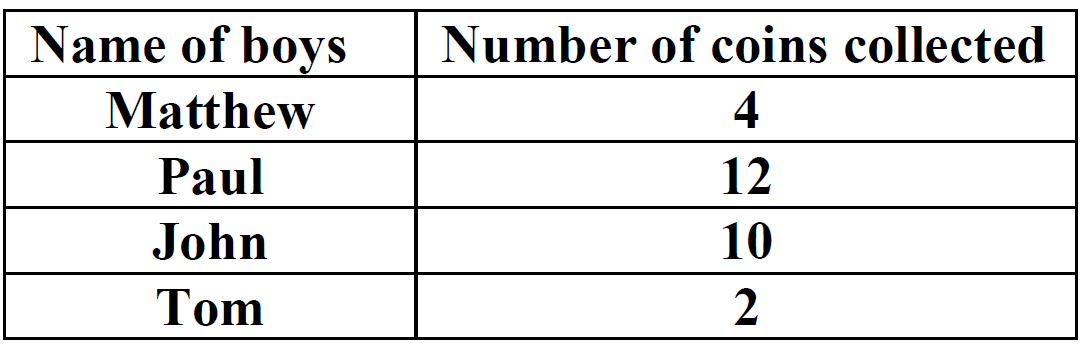#### Examine the picture below. Use it to answer question 56.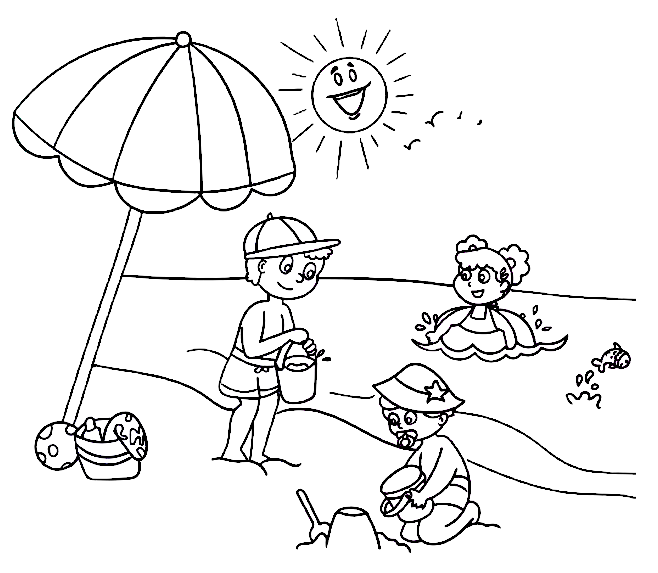#### The diagram below shows two pieces of paper. Use it to answer question 57.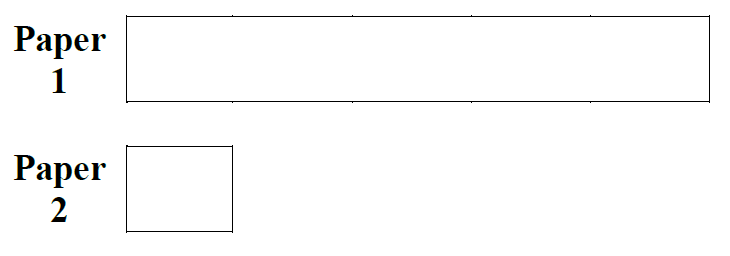#### The diagrams below show a 1-litre jug and a 250 ml bottle. Use them to help you answer question 59.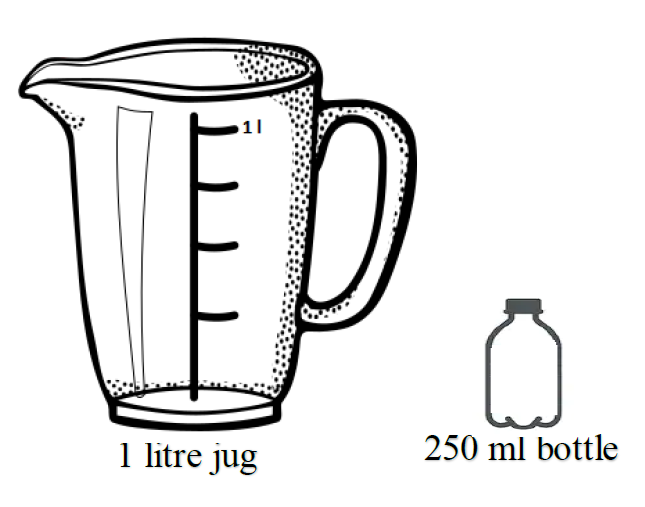#### The diagram below shows the mass of some pencils in grams. Use this information to answer question 60.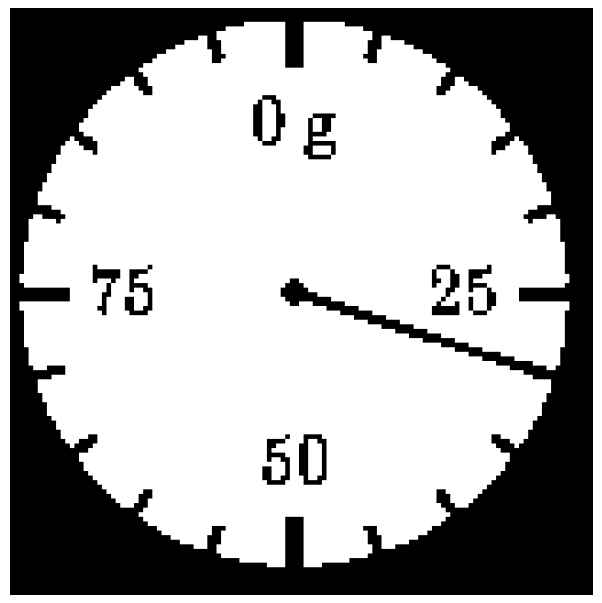Home

# Report

Strand Cluster Number of items Number of responses at each level
level1 level2 level3 level4
Number Set Theory 2
Total /2
Properties of Numbers 4
Total /4
Number Operation – Whole Numbers 5
Total /5
Number Operation – Decimals 3
Total /3
Number Operation – Fractions 7
Total /7
Measurement Using Units of Measurement 2
Total /2
Metric Conversion 3
Total /3
2D Measurement Concepts (e.g. Perimeter and Area) 3
Total /3
Geometry 2D Geometric Concepts 8
Total /8
Algebra Solving Equations and Inequalities 2
Total /2
Patterns 2
Total /2
Statistics and Probability Data Analysis 2
Total /2
Interpreting Statistical Data 2
Total /2
Probability Concepts 2
Total /2
Problem Solving 7
Total /7
Approximation and Estimation 6
Total /6
Total /60